# 1.学习总结(2分)

## 1.1树结构思维导图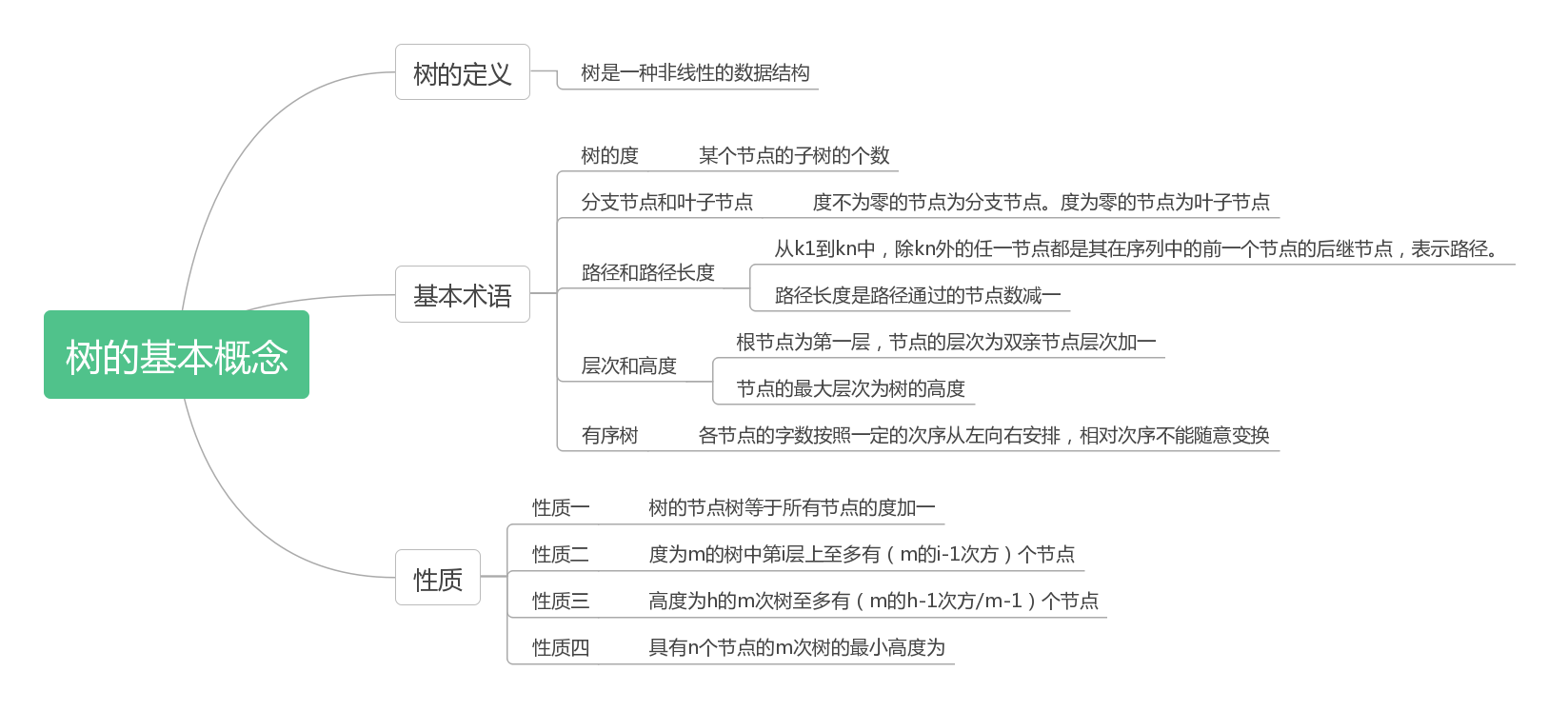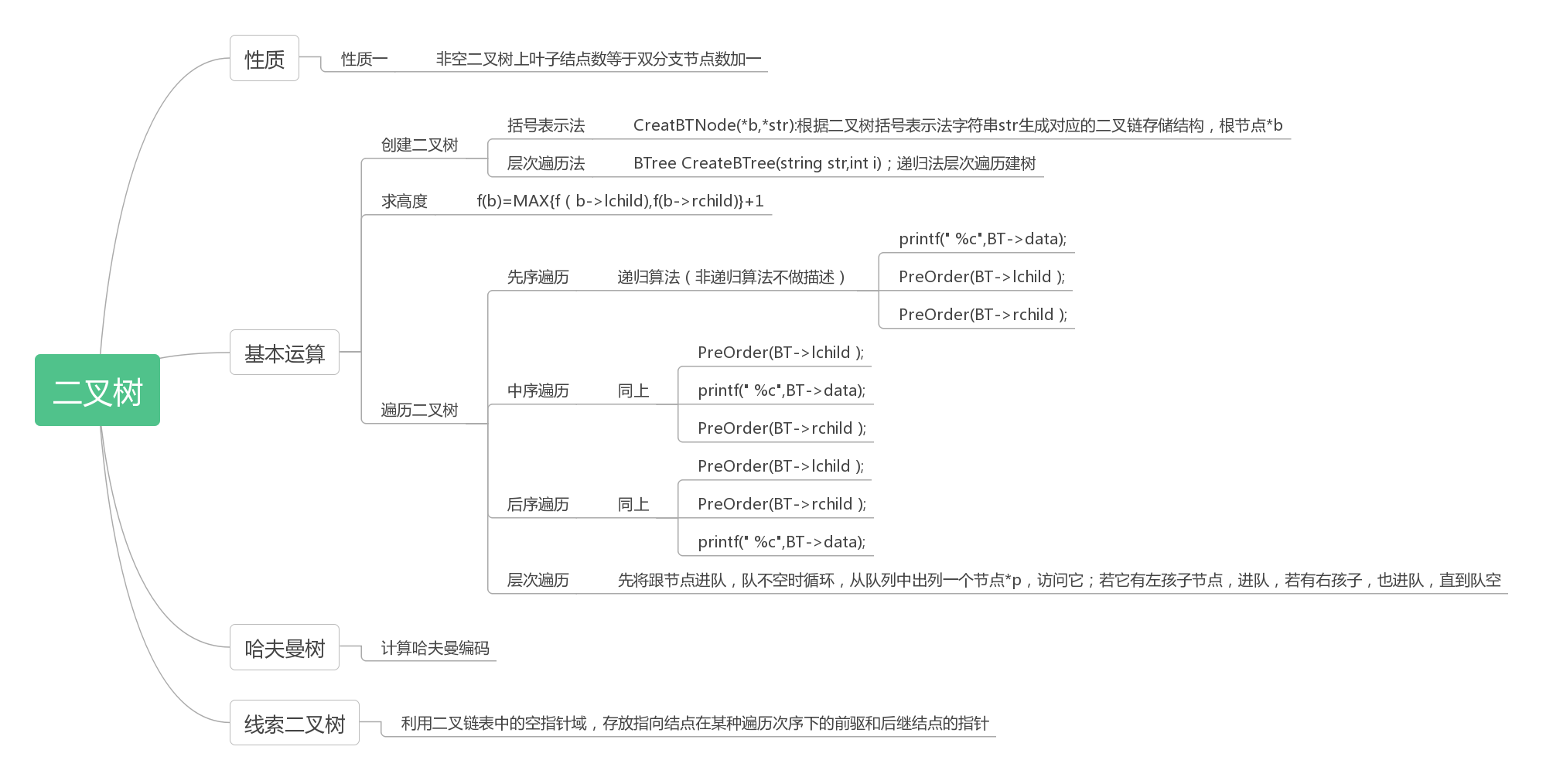# 2.PTA实验作业（4分）

6-4必选

## 2.2 设计思路（伪代码或流程图）

1.先序递归函数
2.空树返回0；
3.if（找到节点的左右孩子都为空，说明为叶子节点）{
输出叶子节点；
}
4.else   {
递归左孩子；
递归右孩子；
}


## 2.3 代码截图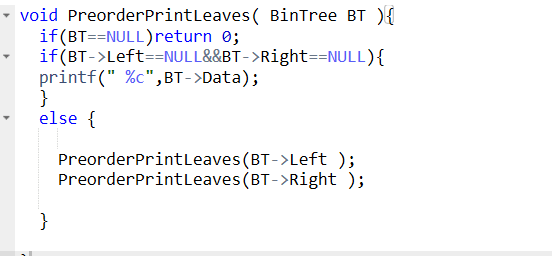## 2.4 PTA提交列表说明。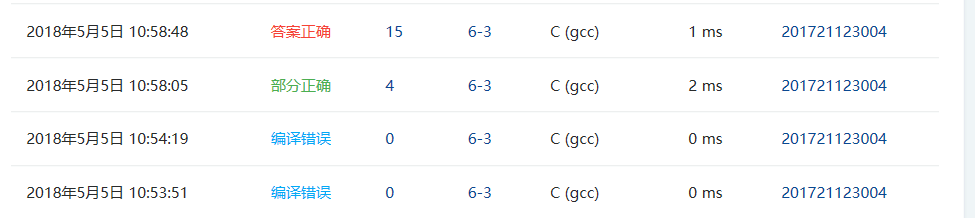## 2.2 设计思路（伪代码或流程图）

1.主函数
2.定义两个字符串并输入；
3.创建树   * s；
4.（创建树的函数）
如果是空树，返回NULL；
for（  int 指针p指向中序序列，遍历中序序列）{
如果指针指向了后序序列post+n+1；退出；
}
定义k=p-in；
递归左孩子（post，in，k）；
递归右孩子（post+k，p+1，n-k-1）；
返回树；
5.先序输出；（函数不写）；


## 2.3 代码截图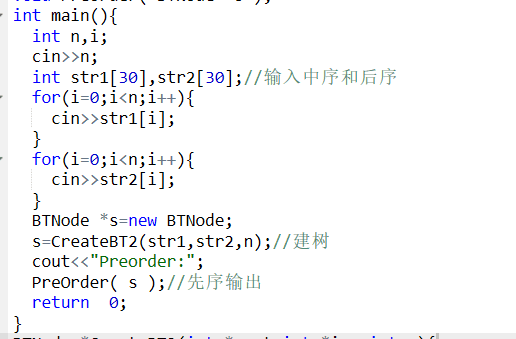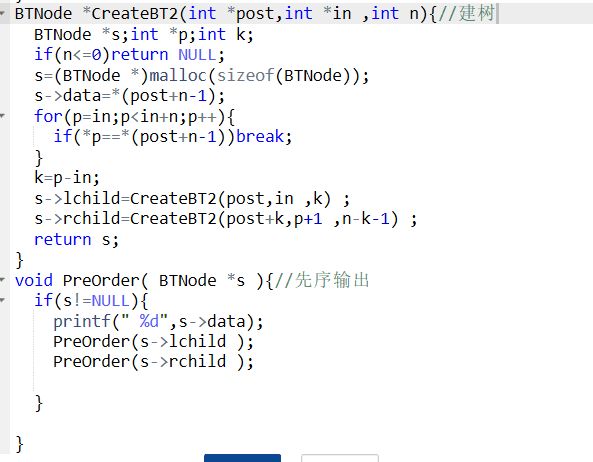## 2.4 PTA提交列表说明。## 2.2 设计思路（伪代码或流程图）

1.定义字符栈和树栈；字符栈先入栈‘#’；
2.for   数组不为空{
如果是数字，存入树栈；
否则，如果是字符，存入字符栈；
此时，如果字符栈已有元素，且字符栈元素优先级大于要存入的字符，将字符出栈和树栈中的数字组成新的树；
如果小于，直接存入字符栈；
其他可能性，出栈；
}for循环结束；
3.  如果字符栈此时不空；
将字符栈元素出栈并和树栈元素组成新的树；
4.计算函数
定义a，b；
树的左右孩子不为空时，返回T->data;
a=递归树的左孩子；
b=递归树的右孩子；
如果是字符元素{
相应计算，不做说明；
除法特别，判断除数是否为0；是的话输出错误；



## 2.3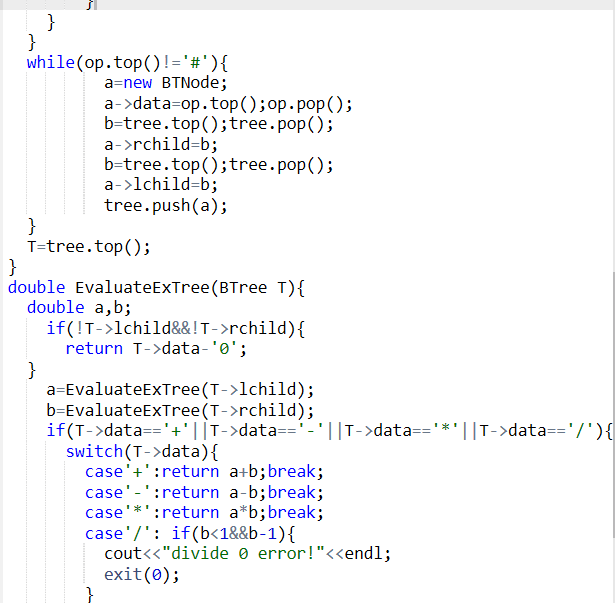## 2.4 PTA提交列表说明。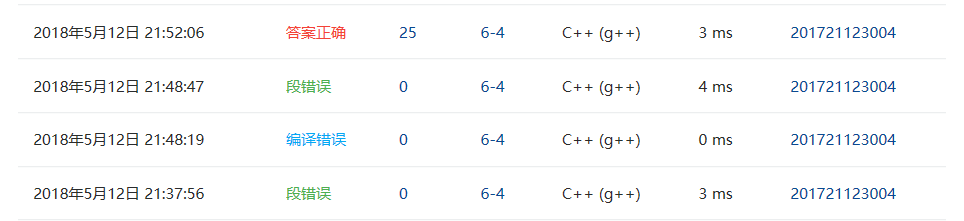# 3.截图本周题目集的PTA最后排名（3分）

## 3.1 PTA排名# 4. 阅读代码（必做，1分）

#include <stdio.h>
#include<stdlib.h>
#include<string.h>
typedef struct node *Node;
struct node {
char Name;
int space;
int  Parant;
};

Node Tree;
int n;

int Scan(char*);
int Trace(int);
int judgeParent(int,int);//父子
int judgeSibling(int,int);//兄弟
int judgeAncestor(int,int);//祖先
void work();
int Index(char*);

int main() {
int m;
scanf("%d%d",&n,&m);
Tree=(Node)malloc(sizeof(struct node)*n);
getchar();//清除缓存
for(int i=0; i<n; i++) {
Tree[i].space=Scan(Tree[i].Name);
Tree[i].Parant=i;
}
Tree.Parant=-1;

for(int i=0; i<m; i++) {
work();
getchar();
}

return 0;
}
int judgeParent(int x,int y) {
if(Tree[x].Parant==x)Tree[x].Parant=Trace(x);
return Tree[x].Parant==y;
}
int judgeSibling(int x,int y) {
if(Tree[x].Parant==x)Tree[x].Parant=Trace(x);
if(Tree[y].Parant==y)Tree[y].Parant=Trace(y);
return Tree[x].Parant==Tree[y].Parant;
}
int judgeAncestor(int x,int y) {
while(x!=-1) {
if(judgeParent(x,y))return 1;
else x=Tree[x].Parant;
}
return 0;
}

void work() {
char StrX,StrY,relation;
scanf("%s%*s%*s%s%*s%s",StrX,relation,StrY);
//  printf("%s - %s - %s\n",StrX,relation,StrY);

int X=Index(StrX);
int Y=Index(StrY);
//  printf("%d   -    %d",X,Y);
int result;
switch(relation) {
case 'c':
result=judgeParent(X,Y);
break;
case 'p':
result=judgeParent(Y,X);
break;
case 's':
result=judgeSibling(X,Y);
break;
case 'd':
result=judgeAncestor(X,Y);
break;
case 'a':
result=judgeAncestor(Y,X);
break;
default:
result=-1;
break;
}

if(result==1)printf("True\n");
else if(!result)printf("False\n");
//  else printf("ERROR:系统不能识别所指定关系！\n");
}

int Index(char*a) {
for(int i=0; i<n; i++) {
//      printf("*");
if(strcmp(Tree[i].Name,a)==0)return i;
}
//  printf("ERROR:所给人名不存在！\n");
return -1;
}

int Trace(int child) { //往前遍历第一个比他缩进少的就是他的父亲

for(int i=child-1; i>=0; i--) {
if(Tree[i].space<Tree[child].space) {
//      printf("%d's parent is %d'",child,i);
return i;
}
}
return -1;//如果没有，那么他就是亚当夏娃了。
}

int Scan(char*p) {
char c;
int space=0;

while((c=getchar())==' ')space++;//记录字符串前面的空格数量

do {
*p++=c;
} while((c=getchar())!='\n');
*p='\0';

return space;
}


# 5. 代码Git提交记录截图posted @ 2018-05-05 20:44  一叶落而秋将至  阅读(229)  评论(2编辑  收藏  举报